# Integration by Parts

Integration by parts is a useful strategy for simplifying some integrals. It is based on the combination rule for differentiation and the general approach can be summarized by: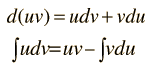This technique is particularly appropriate for removing a linear term multiplying an exponential. For example, the integral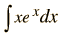can be simplified by the identification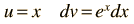Then u can be differentiated and dv can be integrated.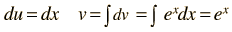The value of the integral is then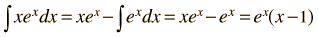Applications
 Average particle energy Mean particle lifetime

If a higher power term of x multiplies the exponential, then the process of integration by parts can be repeated to reduce the power term. Two stages are necessary to integrate a square term.

Example:
 Hydrogen ground state probability

Three stages are necessary if the integrand has a cubic term, and so on.

Example: